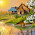## Thursday, December 16, 2010

### Basic Theory on AVR Timer0

To start off with, the formulae relating duty cycle, frequency and time period is: f=1/T, D=t/T where D is duty cycle, T is time period, t is on time, f is frequency
Vo = D * Vin, where Vo = average voltage output from AVR pin, D = duty cycle, Vin = Supply Voltage, eg 5v
The output voltage here is in the form of pulses not a complete analog value.
Eg. When frequency is 100Hz, duty cycle is 50% and supply voltage to AVR is 5v, then output from AVR pin is 2.5v. However, this does not mean that the output will be 2.5v DC. The output will be a pulsating output with frequency = 100Hz, 50% on, 50% off, with high state or on state voltage = 5v, low state or off state voltage = 0v. Therefore output = 2.5v, but actually 5v with 50% on and 50% off. This CAN be converted to pure DC by placing an RC or LC filter, which will convert the pulsating DC to a clean fixed DC voltage.
I will refer to ATMEGA8 for example, as this seems to be one of the most popular AVRs.
There are 3 timers – Timer/Counter 0, Timer/Counter 1 and Timer/Counter 2. I will refer to Timer/Counter as TC, so TC0 means Timer/Counter 0.
TC0 and TC2 are 8-bit timers. This means that the timers will count from 0 to (2^8) – 1 = 255. TC2 has one associated compare module. TC1 is 16-bit, so it counts from 0 to (2^16)-1 = 65535. It has two compare modules attached and provides a rich set of PWM functions.
I’ll start off with TC0.
TC0 has 4 registers associated with it that we need to work with. The most important is Timer/Counter 0 Control Register – TCCR0.

Take a look at the registers below:

The CS2, CS1 and CS0 bits determine the rate at which the value of the TC increments. Say we have a clock of 10MHz. Therefore we have a clock cycle = T = 1/F = 1/10MHz = 100ns. Prescaler extends this time by a specific amount. So, looking at TCCR0, if we write 0 to it, we have no clock source, this means the TC is stopped and will not increment. It retains its current value.
If we set CS[2…0] to 1, we have no prescaler, that means that the TC increments each 100ns, ie each clock cycle. If we assign prescaler 8, by writing 2 to CS[2…0], we extend the time at which TC increments by 8. With no prescaling it would take 1 clock cycle for each increment, therefore with a prescaler of 8, the time would be extended 8 times, ie, it would now take 8 clock cycles for each increment, ie 800 ns.
The same follows for prescaler values of 64, 256 and 1024.
Eg. With a clock cycle of 10MHz, prescaler = 1024, the time taken by TC0 for one increment is:
Time for 1 increment with no prescaling = 1 clock cycle = 1/10MHz = 100ns
Therefore, time for 1 increment with prescaler = 1024 = 1024 clock cycles = 1024*0.1us = 102.4 us
[us = microseconds, ns = nanoseconds]
I will not explain the external clock source as it is not required for PWM.

Take a look at the registers below:

The second associated register is TCNT0 that holds the current value of TC0.
E.g. What will be the value in TCNT0 when CS[2…0] is cleared (=0) after a time of 10secs? Clock = 1MHz, prescaler = 8.
Solution:
Each clock cycle = 1/1MHz = 1us [us = microsecond]
TC0 is incremented (if prescaler = 0) each clock cycle, ie each us(microsecond)
Therefore TC0 is incremented (when prescaler = 0) each 8 clock cycles, ie each 8 us(microsecond)
10 seconds = 10,000,000 microseconds
TC0 counts to a maximum of 255. That’s 255 increments. After this it overflows, ie, it rolls over from TOP [TOP means the maximum value to which TC0 can increment], in this case 255, to 0.
Each increment takes 8 us.
Therefore 256 increments (255 increments + 1 increment to overflow to 0) takes 256 * 8us = 2048us = 2.048ms [ms = milliseconds] = 0.002048 seconds
Therefore in 10 seconds, number of overflows = (10/0.002048) = 4882.8125
That’s 4882 overflows. There’s a remainder, 0.8125, which means a remainder of 0.8125*255 = 207.1875
Therefore 207 is stored in TC0.

Take a look at the registers below:

The third associated register is TIMSK – Timer/Counter Interrupt Mask Register. Here bit 0 is what is associated with TC0. TOIE0 – Timer/Counter Overflow Interrupt Enable 0. Setting this bit enables setting TC0 interrupt. I’ll go into details about this later.

Take a look at the registers below:

The last associated register is TIFR – Timer/Counter Interrupt Flag Register. Here bit 0 is what is associated with TC0. TOV0 = Timer/Counter Overflow Flag 0. This flag is set whenever the timer overflows (this has been shown in the above example). Writing one to this bit clears it.

There's more to come about the Timer PWM modes and Timer1 and Timer2 and all PWM modes.
Hope this is helpful to all.

Registers being referred to:1.2.